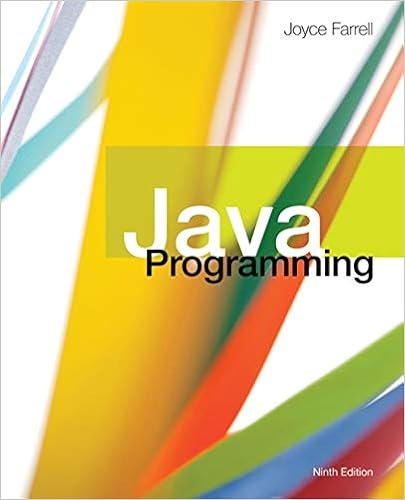# What is the big o running time of the following code

• Homework Help
• 4
• 100% (1) 1 out of 1 people found this document helpful

This preview shows page 2 - 4 out of 4 pages.

##### We have textbook solutions for you!
The document you are viewing contains questions related to this textbook.The document you are viewing contains questions related to this textbook.
Chapter 9 / Exercise 18
Java Programming
FarrellExpert Verified
4. What is the Big-O running time of the following code fragment?Assume lst has N items.public static int calc( List<Integer> lst )
##### We have textbook solutions for you!
The document you are viewing contains questions related to this textbook.The document you are viewing contains questions related to this textbook.
Chapter 9 / Exercise 18
Java Programming
FarrellExpert Verified
{int sum = 0;int N = lst.size();for ( int i=0; i<N; i++){if (lst.get(i) > 0)sum += lst.get(i);elsesum += lst.get(i) * lst.get(i);}return sum;} a. If an ArrayList is passed. Explain your answer.b. If a LinkedList is passed. Explain your answer.
5. Suppose a Java method receives a List<Integer> and reverses the order of the items it contains by removing each item from the front of the list, pushing each item onto a Stack<Integer>, and then popping the items from the stack and inserting each item at the end ofthe list. Assume push and pop are O(1).What is the expected Big-O running time if:a. If an ArrayList is passed. Explain your answer.b. If a LinkedList is passed. Explain your answer.
20 points6. Show each step of converting a+b*c+(d-e) from infix to postfix notation, using the algorithm described in the textbook that uses a stack.
Submit:A file named assignment3.zip.
•••